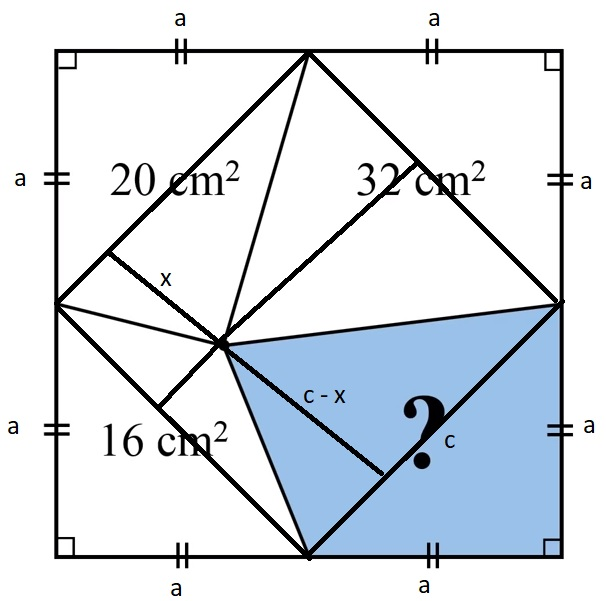Categories

# Another slow solution

Saw this small problem on YouTube, as usual got to the solution but took time + it was not very straight forward like in the video scroll below for it 🙂big total square area = (2a)^2 = 4a^2
corner triangles hipotenuse Pythagorean theorem c^2 = a^2 + a^2 -> c=sqrt(2)a

one corner triangle area = big total square / 8 -> a^2 / 2

20 cm^2 = two triangles area = (a^2 / 2) + (x * sqrt(2)a) / 2
-> a^2 + x * sqrt(2)a – 40 = 0 (1)

blue area n
n = big square area minus 20 minus 32 minus 16
n = 4a^2 – 68
n also two triangles area = (a^2 / 2) + (((sqrt(2)a – x) * sqrt(2)a) / 2
->
2n = 8a^2 – 136 = a^2 + ((sqrt(2)a – x) * sqrt(2)a
7a^2 – 2a^2 + x * sqrt(2)a – 136 = 0
5a^2 + x * sqrt(2)a – 136 = 0 (2)

combining (1) and (2)
5a^2 + x * sqrt(2)a – 136 = a^2 + x * sqrt(2)a – 40

4a^2 = 96
n = 4a^2 – 68
n = 28